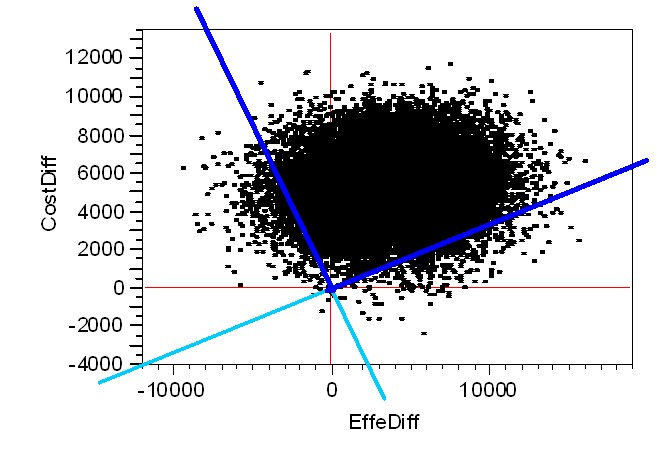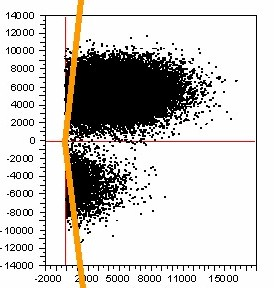### Nav: Back

 Fieller's Theorem: the "original" Wedge-Shaped ICE Confidence Region

Asymptotic Bivariate Normality of ICE Differences

Again, because ICE Inference is based upon sample mean values in Cost and Effectiveness measures, the (multivariate) Central Limit Theorem clearly applies, and the corresponding ICE Differences clearly tend towards having a limiting Bivariate Normal Joint Distribution under rather weak regularity conditions.  Because this assumption of normality is frequently quite realistic, it is extremely natural to use the Fieller(1954) theorem to develop confidence limits for the unknown, true ICE Ratio, (CostDiff divided by EffeDiff).  Naturally, these intervals and the corresponding  BOW-TIE Shaped confidence regions are very different geometrically (and visually)  from the much better-known, elliptical regions needed to "cover" the unknown, true ICE Outcome PAIR, (EffeDiff, CostDiff) with stated confidence!

Application of Fieller's Theorem in ICE Inference

Chaudhary and Stearns (1996) is an important publication in ICE literature primarily because they displayed a closed-form expression for computing the Fieller Confidence Interval ...and its corresponding BOW-TIE Shaped, bivariate confidence region.  Earlier papers by OBrien, Willan and others had explored various, simple Taylors series expansion approximations.

Practical Problems in interpreting Confidence Intervals (Regions) from Fieller's Theorem

[a] Small Numerical Differences really are Rather Un-Important!

Consider the following numerical example:

Fiellers Theorem 95% Interval: -1.7652, +0.3463
ICE Angle Order Statistic 95% Interval: -1.7834, +0.3737

When "too many" decimal places are reported (as in the above numerical listings), the Fieller Interval may appear to be "different" from that of the corresponding Bootstrap ICE Angle Interval.  However, the graphical display below of the corresponding 2-dimensional Confidence Regions  clearly shows that the Fieller and Bootstrap approaches can be essentially equivalent in many cases...[b] Problematic "BOW-TIE" Shape:  Which "Half" should be Thrown Away?

In the above graphic, the "top half" of the Fieller BOW-TIE (that spans parts of the North-West and North-East ICE Quadrants) is clearly the part to "keep."  After all, this particular "wedge" contains not only the ICE Outcome Pair, (+1,900, +5,289), of Observed (Effectiveness, Cost) Differences but also roughly 95% of the Bootstrap Re-sampled Outcomes!  The "bottom half" (that spans parts of the South-West and South-East ICE Quadrants) has the same (finite, polar angular) size but contains almost no Bootstrap Re-sampled Outcomes.  And the two remaining wedges each contains only roughly 2.5% of the Bootstrap Re-sampled Outcomes.

In summary, it usually is rather easy to decide which parts of the Fieller BOW-TIE to throw away and ,thus, which single WEDGE to KEEP!  Still this sort of additional processing is a (mild) hassle in the sense that it always needs to be done!

[c] "Infinite Intervals" can Result!

In fact, this phenomenon occurred in the above example!  The Fieller Interval (as well as the corresponding ICE Angle Interval) contain all ICE Rays with Negative Slopes in the North-West Quadrant that are numerically smaller (more negative) than roughly -1.77 as well as all ICE Rays with Positive Slopes in the North-East Quadrant that are numerically larger (more positive) than roughly +0.35.  In other words, both the Fieller and Bootstrap intervals for this example can be described as consisting of the half-open interval (minus infinity, -1.77] plus the half-open interval [+0.35, plus infinity)  ...with both parts being infinite in length!

I really do not know why several ICE Confidence Interval "critics" have tried to make such a BIG DEAL out of this phenomenon.  The reality is that ICE Ratios (Slopes) have a simple discontinuity along the Vertical (EffiDiff=0) Axis ...where they suddenly jump between plus infinity and minus infinity.  My advise is this: Do not freak-out novices by reporting these sorts of Intervals numerically; again, simply display the corresponding Confidence Region graphically!

[d] Fieller's Theorem "Explodes" in High Uncertainty Cases!

Oh, Oh!  This really is a MAJOR SHORTCOMING.  In fact, this is the ONLY REASON why the Bootstrap Re-sampling approach using ICE Angle Order Statistics should be considered to be more stable, robust and realistic than the Fieller's Theorem approach!

By the way, it's rather obvious what goes "WRONG" with the Fieller BOW-TIE in these "high uncertainty" cases.  Each of the opposing wedge-shaped "halves" of the BOW-TIE are of the same (angular) size, so neither half can be wider than 180-degrees!!!  Thus, as the ICE Radius of the center-point of the Bootstrap Re-sampling scatter decreases and the scatter approaches (and its Convex-Hull ultimately covers) the ICE Origin, (0, 0), the Fieller BOW-TIE WIDENS and eventually attempts to COVER the entire ICE Plane.  Technically, the quantity within the square-root in the closed-form expression of Chaudhary and Stearns (1996) then becomes NEGATIVE, and the only corresponding "solutions" for Fieller Confidence Limits become IMAGINARY!

In stark contrast, this sort of "explosion" simply cannot happen in the Bootstrap Re-sampling approach using ICE Angle Order Statistics because the Central Polar Angle of the Bootstrap Wedge can easily exceed 180-degrees!  In fact, the example wedge displayed on our very first ICE Tutorial Page had a Central Polar Angle spanning 237-degrees (i.e. considerably more than HALF of the full Bootstrap Re-sampling "PIE.")

Misconceptions that have lead to Highly Questionable Simulation Results

[a] Meaningless "Order Statistics" can result from sorting ICE Ratios instead of ICE Angles

The following is a computational "blunder" that I have seen being repeated over and over again (in both ICE applications and ICE simulation studies.)  It's simply not appropriate (and can be incredibly misleading and biased) to (i) calculate the ICE Ratio for each Bootstrap Re-sample Outcome, to then (ii) sort these values from numerically largest (most positive) to numerically smallest (most negative), and finally (iii) to form a 95% Interval by "Counting Inwards" 2.5% of these ICE Ratio Order Statistics from each supposed "end" of the resulting distribution.

This faulty algorithm essentially makes the incredibly naive assumption, (depicted visually below) that all Bootstrap Re-sampled Outcomes always have an observed EffeDiff that is non-negative!  LOOK how "distorted" and "disjointed" the actual Bootstrap Distribution (depicted above) becomes due to this blunder ...and how LARGE and incredibly BIASED this blunder can make the resulting ICE Ratio confidence wedge!!!ICE Ratio Order Statistic Interval: +12.4343, -12.458

In my opinion, all published simulation studies that claim that bootstrap ICE intervals are "biased" (or have "inferior" coverage) relative to Fieller ICE intervals make this same, incredibly naive computational mistake.

[b] The ICE Ray with the Most Positive ICE Slope does not necessarily correspond to the Upper (Counter-Clockwise) Confidence Limit

We also saw this phenomenon in the above numerical example!  The correct Counter-Clockwise (Upper) Confidence Limit was the negative value (of -1.77 or -1.78 within the SW Quadrant) rather than the positive value (of +0.35 or +0.37 within the NE Quadrant.)

[c] Incorrect or Misinterpreted Trignometric Formulas

I think that the simulation results reported in Cook and Heyse (2000) are credible; anyway, they do agree with what I have found in my own ICE experience.  On the other hand, one of their formulas does not give correct answers in any computing environment that I have tried it in:

Theta (degrees)  = 180*atan(DelCost/DelEffe)/pi
+ 180 when DelCost is negative

Unfortunately, Fan and Zhou (2007) apparently trusted this formula and (not surprisingly) got simulation results that conflict with those of Cook and Heyse (2000)!!!

The following alternative formula gives correct answers in the "R" environment:

Theta (degrees)  = 180*atan(DelCost/DelEffe)/pi
+ 180 when DelCost is negative
+ 180 when DelEffe * DelCost is negative

The JMP (from SAS) environment returns a "missing value" when asked to compute atan(1/0) and atan(-1/0).

WARNING:  Obenchain manuscripts emphasize the ICE Preference Symmetry Axiom and, thus, place the ICE Angle Origin (Theta = 0) along the x = -y diagonal within the SE ICE Quadrant.  Correspondingly, I also restrict attention to the finite ICE Angle range of (-180, +180] degrees.  Cook and Heyse (2000) place their Theta origin at my Theta = +45 degrees.  Furthermore, they essentially use the "positive" Theta range of [0, 360) degrees.

Summary

ICE Inference practitioners really do need to be discouraged from using computing  algorithms that have not been validated.  If nothing else, a suite of benchmark numerical examples and corresponding "correct" analyzes needs to be established to speed testing of all new/alternative algorithms.    Furthermore, consensus on what (objectively) really are the relative strengths and weaknesses of alternative ICE methodologies is quite badly needed.  My very first ICE communication (1997) called for collaboration on these vital sorts of "issues and algorithms."

References:

Fieller EC.  Some problems in interval estimation. J R Stat Soc B 1954; 16, 175-183.

OBrien BJ, Drummond MF, Labelle RJ, Willan A.  In search of power and significance: issues in the design and analysis of stochastic cost-effectiveness studies in health care.  Med Care  1994; 32: 150-163.

Willan AR, O'Brien BJ.  Confidence intervals for cost-effectiveness ratios: an application of Fieller's theorem.  Health Economics 1996; 5:297-305.

Stinnett AA.  Adjusting for bias in C=E ratio estimates.  Health Economics 1996; 5:470-472.

Chaudhary MA, Stearns SC.  Estimating confidence intervals for cost-effectiveness ratios: an example from a randomized trial.  Stat Med 1996; 15: 1447-1458.

Laska EM, Meisner M, Siegel C.  Statistical inference for cost-effectiveness ratios.  Health Economics 1997; 6: 229-242.

Polsky D, Glick HA, Willke R, Schulman K.  Confidence intervals for cost-effectiveness ratios: a comparison of four methods.  Health Economics  1997; 6: 243-252.

Tambour M, Zethraeus N. Bootstrap confidence intervals for cost-effectiveness ratios: some simulation results. Health Economics  1998; 7:143-147.

Briggs AH, Fenn P.  Confidence intervals or surfaces?  Uncertainty on the cost-effectiveness plane (Student Corner.)  Health Economics 1998; 7: 723-740.

Briggs AH, Mooney CZ, Wonderling DE.  Constructing confidence intervals for cost-effectiveness ratios: an evaluation of parametric and non-parametric techniques using Monte Carlo simulation.  Stat Med  1999; 18: 3245-3262.

Cook JR, Heyse JF.  Use of an angular transformation for ratio estimation in cost-effectiveness analysis.  Stat Med  2000; 19: 2989-3003.

Jiang G, Wu J, Williams GR.  Fiellers interval and the bootstrap-fieller interval for the incremental cost-effectiveness ratio. Health Serv Outcomes Res Method  2000; 1: 291-303.

Fan MY, Zhou XH.  A simulation study to compare methods for constructing confidence intervals for the incremental cost-effectiveness ratio.  Health Serv Outcomes Res Method 2007; 7: 57-77.